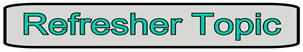Like Terms MathBitsNotebook.com Terms of Use   Contact Person: Donna RobertsMonomials that have the same variable factors are called like terms.

In plain English, these are terms whose variables (including the exponents) are the same. They are terms that look like each other. The only difference will be the coefficients (the numbers in front of the variables).

 Like Terms: Notes: 3, 12, -4, 5x0 The simplest "like terms" are numbers (constants). Remember that the "variable" in a constant term has an exponent of 0, making the variable equal to 1. 3x2 , 6x2 , -2x2 , x2 These are "like terms" because each has the variable factor of x2 . 4a2b3, a2b3, -3a2b3 These are "like terms" because each has the variable factor of a2b3. Not Like Terms: Notes: 3, 4xy, -2a, 5x2y These terms do not have the same variable factor. Remember that xy and x2y are different terms. These examples are unlike terms.

Like terms can be combined under addition.
This process is the same as adding apples to apples, and oranges to oranges.
Just remember to add only the terms that are "like".

 Problem: Answer: • Simplify: 2x + 4x + x Don't forget the implied "1" in front of x. Think of it as two x's plus four more x's plus one more x. You can also factor: x(2 + 4 + 1) = 7x Answer: 7x • Simplify: 2x2 + x - 5 + x2 - 4x + 9 Group the like terms together and them combine them. Remember when moving terms to move their signs with them. 2x2 + x2 + x - 4x - 5 + 9 Factor: x2 (2 + 1) + x (1 - 4) - 5 + 9 Answer: 3x2 - 3x + 4 • Simplify: 6x2 - (10 + 3x2 - 2x) Deal with parentheses before you combine the like terms. In this case, distribute -1 across the parentheses. 6x2 -1(10 + 3x2 - 2x) 6x2 -10 - 3x2 + 2x = 6x2 - 3x2 + 2x -10 Answer: 3x2 + 2x -10 • Simplify: a2b + 2(3a2b - 5a) Distribute the +2 across the parentheses. a2b + 2(3a2b - 5a) = a2b + 6a2b - 10a Answer: 7a2b - 10a • Simplify: 5x2 - 4x3 - x2 + 8 Look carefully at the exponents. Answer: - 4x3 + 4 x2 + 8